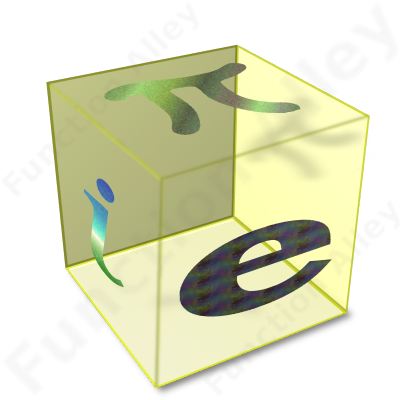# The Factory

This web-site isn't primarily about maths, it's more about functional-programming & Haskell; maths is merely the current focus of my short attention-span. The result is that I've implemented several algorithms in each of the following domains, most of which concern number-theory (a senseless homographic pun), but I've left the mathematical description to those bald enough to know better. The functions I've implemented are intended to form an API, but have also been linked into an application, to facilitate measurement of their performance. The following modules are the most significant in the package "factory":To support these, there are several ancillary modules:

Arithmetic-geometric mean:

To support the Brent-Salamin algorithm for Pi.

Hyperoperations:

The Ackermann-Péter function, tetration, pentation …

Polynomial:

Supports basic () polynomial arithmetic, including division, & exponentiation, as required to support the AKS primality-test.

Power:

Implements algorithms for fast modular exponentiation by squaring, & to detect square numbers & perfect powers.

Radix:

Permitting the expression of integers in alternative number-systems, including support for negative bases.

Probability:

Generation of sample populations with either Normal or Poisson distribution.

Statistics:

Calculation of; mean, variance, standard-deviation, nCr, nPr.